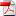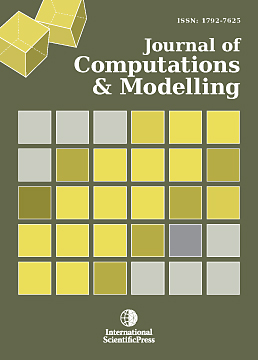# Journal of Computations & Modelling

#### Splitting Approach to Coupled Navier-Stokes and Molecular Dynamics Equations

•[ Download ]
• Abstract

In this paper, we present a multi-scale method with a splitting approach based on iterative operator splitting methods, which takes into account the disparity of the macro- and microscopic scales. We couple the Navier–Stokes and the Molecular Dynamics equations, while taking into account their underlying scales. The underlying ideas are to save computational costs by decoupling complicated systems. Combining relaxation methods and averaging techniques we can optimize the computational effort. The motivation arose from modeling fluid transport under the influence of a multiscale problem, which has to be solved with smaller time scales, e.g., non-Newtonian flow problem. The applications include colloid damper or fluid–solid problems, where we study an area where the Navier–Stokes equations have less information about the stream field and we need at least the Boltzmann equation to obtain enough information about the whole density field. A novel research field is, e.g., Carbon Nanotubes, where we have to couple macro- and micromodels and obtain a fluid–solid area which uses the Lennard–Jones fluid model. The proposed method for solving such delicate problems enables simulations in which the continuum flow aspects of the flow are described by the Navier–Stokes equations at time-scales appropriate for this level of modeling, while the viscous stresses within the Navier–Stokes equations are the result of Molecular Dynamics simulations, with much smaller time-scales. The main benefit of the proposed method is that the time dependent flows can then be modeled with a computational effort which is significantly smaller than if the complete flow were to be modeled at the molecular level, as a result of the different time-scales at the continuum and molecular levels, enabled by the application of the iterative operator-splitting method. We discuss the convergence analysis of these splitting methods, see also . Finally we present numerical results for the modified methods and applications to real-life flow problems.ISSN: 1792-8850(Online)
1792-7625 (Print)